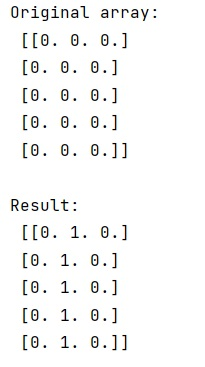# Python - Assign 2D NumPy array column value as the values of the 1D array

Learn, how to assign 2D NumPy array column value as the values of the 1D NumPy array in Python?
Submitted by Pranit Sharma, on March 25, 2023

## Assigning to columns in NumPy

Suppose that we are given a 2D numpy array with multiple rows and columns and with a 1D numpy array. What we need to do is to set a particular column value as the values of the 1D numpy array.

For example, if we are given with a 2D numpy array [[1,0],[3,0]] and a 1D array as [2,4]. We need to replace the second column with the values of array so that it becomes [[1,2],[3,4]].

For assigning to columns in NumPy, the fancy indexing of numpy will work. We need to select the arr[:, 1] to select the second column and x[:,0] for x as a single numpy array.

Let us understand with the help of an example,

## Python code to assign 2D NumPy array column value as the values of the 1D array

```# Import numpy
import numpy as np

# Creating a 2D numpy array
arr = np.array([[ 0.,  0.,  0.],[ 0.,  0.,  0.],[ 0.,  0.,  0.],[ 0.,  0.,  0.],[ 0.,  0.,  0.]])

# Display original array
print("Original array:\n",arr,"\n")

# Creating a 1D array
x = np.ones(5)

# Assigning second column of array with values of x
arr[:,1] = x

# Display result
print("Result:\n",arr)
```

### Output